# Interactive Rigid Objects

This tutorial demonstrates rigid object interactions in Habitat-sim -- instancing, dynamic simulation, and kinematic manipulation.

The example code below is available on Collab, or runnable via:

`\$ python path/to/habitat-sim/examples/tutorials/nb_python/rigid_object_tutorial.py`

Import necessary modules, define some convenience functions, and initialize the Simulator and Agent.

```import math
import os
import random
import sys

import git
import magnum as mn
import numpy as np

import habitat_sim
from habitat_sim.utils import viz_utils as vut

os.environ["IMAGEIO_FFMPEG_EXE"] = "/usr/bin/ffmpeg"

repo = git.Repo(".", search_parent_directories=True)
dir_path = repo.working_tree_dir
# %cd \$dir_path
data_path = os.path.join(dir_path, "data")
output_path = os.path.join(dir_path, "examples/tutorials/rigid_object_tutorial_output/")

def remove_all_objects(sim):
for id_ in sim.get_existing_object_ids():
sim.remove_object(id_)

def place_agent(sim):
# place our agent in the scene
agent_state = habitat_sim.AgentState()
agent_state.position = [-0.15, -0.7, 1.0]
agent_state.rotation = np.quaternion(-0.83147, 0, 0.55557, 0)
agent = sim.initialize_agent(0, agent_state)
return agent.scene_node.transformation_matrix()

def make_configuration():
# simulator configuration
backend_cfg = habitat_sim.SimulatorConfiguration()
backend_cfg.scene_id = os.path.join(
data_path, "scene_datasets/habitat-test-scenes/apartment_1.glb"
)
assert os.path.exists(backend_cfg.scene_id)
backend_cfg.enable_physics = True

# sensor configurations
# Note: all sensors must have the same resolution
# setup 2 rgb sensors for 1st and 3rd person views
camera_resolution = [544, 720]
sensor_specs = []

rgba_camera_1stperson_spec = habitat_sim.CameraSensorSpec()
rgba_camera_1stperson_spec.uuid = "rgba_camera_1stperson"
rgba_camera_1stperson_spec.sensor_type = habitat_sim.SensorType.COLOR
rgba_camera_1stperson_spec.resolution = camera_resolution
rgba_camera_1stperson_spec.postition = [0.0, 0.6, 0.0]
rgba_camera_1stperson_spec.orientation = [0.0, 0.0, 0.0]
rgba_camera_1stperson_spec.sensor_subtype = habitat_sim.SensorSubType.PINHOLE
sensor_specs.append(rgba_camera_1stperson_spec)

depth_camera_1stperson_spec = habitat_sim.CameraSensorSpec()
depth_camera_1stperson_spec.uuid = "depth_camera_1stperson"
depth_camera_1stperson_spec.sensor_type = habitat_sim.SensorType.DEPTH
depth_camera_1stperson_spec.resolution = camera_resolution
depth_camera_1stperson_spec.postition = [0.0, 0.6, 0.0]
depth_camera_1stperson_spec.orientation = [0.0, 0.0, 0.0]
depth_camera_1stperson_spec.sensor_subtype = habitat_sim.SensorSubType.PINHOLE
sensor_specs.append(depth_camera_1stperson_spec)

rgba_camera_3rdperson_spec = habitat_sim.CameraSensorSpec()
rgba_camera_3rdperson_spec.uuid = "rgba_camera_3rdperson"
rgba_camera_3rdperson_spec.sensor_type = habitat_sim.SensorType.COLOR
rgba_camera_3rdperson_spec.resolution = camera_resolution
rgba_camera_3rdperson_spec.postition = [0.0, 1.0, 0.3]
rgba_camera_3rdperson_spec.orientation = [-45, 0.0, 0.0]
rgba_camera_3rdperson_spec.sensor_subtype = habitat_sim.SensorSubType.PINHOLE
sensor_specs.append(rgba_camera_3rdperson_spec)

# agent configuration
agent_cfg = habitat_sim.agent.AgentConfiguration()
agent_cfg.sensor_specifications = sensor_specs

return habitat_sim.Configuration(backend_cfg, [agent_cfg])

def simulate(sim, dt=1.0, get_frames=True):
# simulate dt seconds at 60Hz to the nearest fixed timestep
print("Simulating " + str(dt) + " world seconds.")
observations = []
start_time = sim.get_world_time()
while sim.get_world_time() < start_time + dt:
sim.step_physics(1.0 / 60.0)
if get_frames:
observations.append(sim.get_sensor_observations())

return observations```
```    # create the simulators AND resets the simulator

cfg = make_configuration()
try:  # Got to make initialization idiot proof
sim.close()
except NameError:
pass
sim = habitat_sim.Simulator(cfg)
agent_transform = place_agent(sim)

# get the primitive assets attributes manager
prim_templates_mgr = sim.get_asset_template_manager()

# get the physics object attributes manager
obj_templates_mgr = sim.get_object_template_manager()```

## Simulation Quickstart

Basic rigid body simulation can be achieved by simply loading a template, instancing an object, and stepping the physical world. In this example, a sphere object template is loaded and the object is instanced in the scene above the table. When the simulation is stepped, it falls under the force of gravity and reacts to collisions with the scene.

```    # load some object templates from configuration files
str(os.path.join(data_path, "test_assets/objects/sphere"))
)

# add an object to the scene
sim.set_translation(np.array([2.50, 0, 0.2]), id_1)

# simulate
observations = simulate(sim, dt=1.5, get_frames=make_video)

if make_video:
vut.make_video(
observations,
"rgba_camera_1stperson",
"color",
output_path + "sim_basics",
open_vid=show_video,
)```Forces and torques can be applied to the object with Simulator.apply_force() and Simulator.apply_torque(). Instantanious initial velocities can also be set with Simulator.set_linear_velocity() and Simulator.set_angular_velocity().

In the example below, a constant anti-gravity force is applied to the boxes’ centers of mass (COM) causing them to float in the air. A constant torque is also applied which gradually increases the angular velocity of the boxes. A sphere is then thrown at the boxes by applying an initial velocity.

Note that forces and torques are treated as constant within each call to Simulator.step_physics() and are cleared afterward.

```    observations = []
# search for an object template by key sub-string
cheezit_template_handle = obj_templates_mgr.get_template_handles(
"data/objects/cheezit"
)
box_positions = [
np.array([2.39, -0.37, 0]),
np.array([2.39, -0.64, 0]),
np.array([2.39, -0.91, 0]),
np.array([2.39, -0.64, -0.22]),
np.array([2.39, -0.64, 0.22]),
]
box_orientation = mn.Quaternion.rotation(
mn.Rad(math.pi / 2.0), np.array([-1.0, 0, 0])
)
# instance and place the boxes
box_ids = []
for b in range(5):
sim.set_translation(box_positions[b], box_ids[b])
sim.set_rotation(box_orientation, box_ids[b])

# get the object's initialization attributes (all boxes initialized with same mass)
object_init_template = sim.get_object_initialization_template(box_ids)
# anti-gravity force f=m(-g)
anti_grav_force = -1.0 * sim.get_gravity() * object_init_template.mass

# throw a sphere at the boxes from the agent position
sphere_template = obj_templates_mgr.get_template_by_id(sphere_template_id)
sphere_template.scale = np.array([0.5, 0.5, 0.5])
obj_templates_mgr.register_template(sphere_template)

sim.set_translation(
sim.agents.get_state().position + np.array([0, 1.0, 0]), sphere_id
)
# get the vector from the sphere to a box
target_direction = sim.get_translation(box_ids) - sim.get_translation(sphere_id)
# apply an initial velocity for one step
sim.set_linear_velocity(target_direction * 5, sphere_id)
sim.set_angular_velocity(np.array([0, -1.0, 0]), sphere_id)

start_time = sim.get_world_time()
dt = 3.0
while sim.get_world_time() < start_time + dt:
# set forces/torques before stepping the world
for box_id in box_ids:
sim.apply_force(anti_grav_force, np.array([0, 0.0, 0]), box_id)
sim.apply_torque(np.array([0, 0.01, 0]), box_id)
sim.step_physics(1.0 / 60.0)
observations.append(sim.get_sensor_observations())

if make_video:
vut.make_video(
observations,
"rgba_camera_1stperson",
"color",
output_path + "dynamic_control",
open_vid=show_video,
)```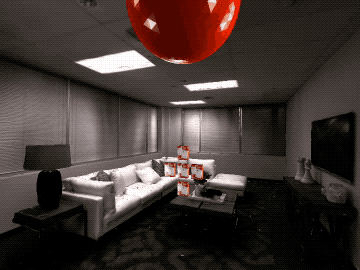## Kinematic Object Placement

Often it is enough to set the desired state of an object directly. In these cases the computational overhead of running full dynamic simulation may not be necessary to achieve a desired result. Setting the object to habitat_sim.physics.MotionType.KINEMATIC with Simulator.set_object_motion_type() specifies that the object’s state will be directly controlled.

In the example below, a kinematic can is placed in the scene which will not react to physical events such as collision with dynamically simulated objects. However, it will still act as a collision object for other scene objects as in the following example.

```    chefcan_template_handle = obj_templates_mgr.get_template_handles(
"data/objects/chefcan"
)
sim.set_translation(np.array([2.4, -0.64, 0]), id_1)
# set one object to kinematic
sim.set_object_motion_type(habitat_sim.physics.MotionType.KINEMATIC, id_1)

# drop some dynamic objects
sim.set_translation(np.array([2.4, -0.64, 0.28]), id_2)
sim.set_translation(np.array([2.4, -0.64, -0.28]), id_3)
sim.set_translation(np.array([2.4, -0.3, 0]), id_4)

# simulate
observations = simulate(sim, dt=1.5, get_frames=True)

if make_video:
vut.make_video(
observations,
"rgba_camera_1stperson",
"color",
output_path + "kinematic_interactions",
open_vid=show_video,
)```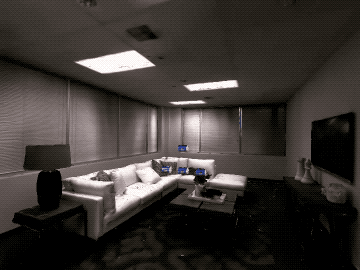## Kinematic Velocity Control

To move a kinematic object, the state can be set directly before each simulation step. This is useful for synchronizing the simulation state of objects to a known state such as a dataset trajectory, input device, or motion capture.

```    observations = []

clamp_template_handle = obj_templates_mgr.get_template_handles(
"data/objects/largeclamp"
)
sim.set_object_motion_type(habitat_sim.physics.MotionType.KINEMATIC, id_1)
sim.set_translation(np.array([0.8, 0, 0.5]), id_1)

start_time = sim.get_world_time()
dt = 1.0
while sim.get_world_time() < start_time + dt:
# manually control the object's kinematic state
sim.set_translation(sim.get_translation(id_1) + np.array([0, 0, 0.01]), id_1)
sim.set_rotation(
* sim.get_rotation(id_1),
id_1,
)
sim.step_physics(1.0 / 60.0)
observations.append(sim.get_sensor_observations())

if make_video:
vut.make_video(
observations,
"rgba_camera_1stperson",
"color",
output_path + "kinematic_update",
open_vid=show_video,
)```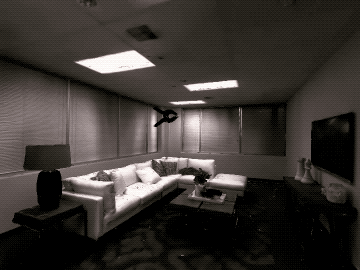However, when applying model or algorithmic control it is more convenient to specify a constant linear and angular velocity for the object which will be simulated without manual integration. The object’s habitat_sim.physics.VelocityControl structure provides this functionality and can be acquired via Simulator.get_object_velocity_control(). Once paramters are set, control takes affect immediately on the next simulation step as shown in the following example.

```    # get object VelocityControl structure and setup control
vel_control = sim.get_object_velocity_control(id_1)
vel_control.linear_velocity = np.array([0, 0, -1.0])
vel_control.angular_velocity = np.array([4.0, 0, 0])
vel_control.controlling_lin_vel = True
vel_control.controlling_ang_vel = True

observations = simulate(sim, dt=1.0, get_frames=True)

# reverse linear direction
vel_control.linear_velocity = np.array([0, 0, 1.0])

observations += simulate(sim, dt=1.0, get_frames=True)

if make_video:
vut.make_video(
observations,
"rgba_camera_1stperson",
"color",
output_path + "velocity_control",
open_vid=show_video,
)```Velocities can also be specified in the local space of the object to easily apply velocity control for continuous agent actions.

```    vel_control.linear_velocity = np.array([0, 0, 2.3])
vel_control.angular_velocity = np.array([-4.3, 0.0, 0])
vel_control.lin_vel_is_local = True
vel_control.ang_vel_is_local = True

observations = simulate(sim, dt=1.5, get_frames=True)

# video rendering
if make_video:
vut.make_video(
observations,
"rgba_camera_1stperson",
"color",
output_path + "local_velocity_control",
open_vid=show_video,
)```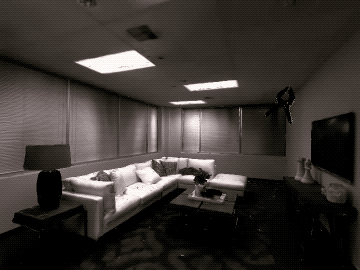## Embodied Agents

For this tutorial section, you will need to download the merged locobot asset and extract it into path/to/habitat-sim/data/objects/

Previous stages of this tutorial have covered adding objects to the world and manipulating them by setting positions, velocity, forces, and torques. In all of these examples, the agent has been a passive onlooker observing the scene. However, the agent can also be attached to a simulated object for embodiement and control. This can be done by passing the Agent’s scene node to the Simulator.add_object() function.

In this example, the agent is embodied by a rigid robot asset and the habitat_sim.physics.VelocityControl structure is used to control the robot’s actions.

```    # load the lobot_merged asset
str(os.path.join(data_path, "objects/locobot_merged"))
)

# add robot object to the scene with the agent/camera SceneNode attached
sim.set_translation(np.array([1.75, -1.02, 0.4]), id_1)

vel_control = sim.get_object_velocity_control(id_1)
vel_control.linear_velocity = np.array([0, 0, -1.0])
vel_control.angular_velocity = np.array([0.0, 2.0, 0])

# simulate robot dropping into place
observations = simulate(sim, dt=1.5, get_frames=make_video)

vel_control.controlling_lin_vel = True
vel_control.controlling_ang_vel = True
vel_control.lin_vel_is_local = True
vel_control.ang_vel_is_local = True

# simulate forward and turn
observations += simulate(sim, dt=1.0, get_frames=make_video)

vel_control.controlling_lin_vel = False
vel_control.angular_velocity = np.array([0.0, 1.0, 0])

# simulate turn only
observations += simulate(sim, dt=1.5, get_frames=make_video)

vel_control.angular_velocity = np.array([0.0, 0.0, 0])
vel_control.controlling_lin_vel = True
vel_control.controlling_ang_vel = True

# simulate forward only with damped angular velocity (reset angular velocity to 0 after each step)
observations += simulate(sim, dt=1.0, get_frames=make_video)

vel_control.angular_velocity = np.array([0.0, -1.25, 0])

# simulate forward and turn
observations += simulate(sim, dt=2.0, get_frames=make_video)

vel_control.controlling_ang_vel = False
vel_control.controlling_lin_vel = False

# simulate settling
observations += simulate(sim, dt=3.0, get_frames=make_video)

# remove the agent's body while preserving the SceneNode
sim.remove_object(id_1, False)

# video rendering with embedded 1st person view
if make_video:
vut.make_video(
observations,
"rgba_camera_1stperson",
"color",
output_path + "robot_control",
open_vid=show_video,
)```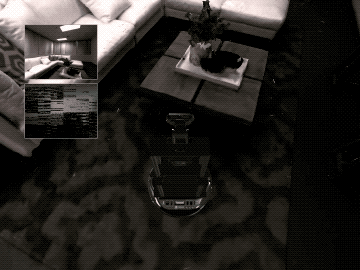## Continuous Control on NavMesh

For this tutorial section, if you have not done so, you will need to download the merged locobot asset and extract it into path/to/habitat-sim/data/objects/

Instead of full dynamic simulation, kinematic state setting and the NavMesh can be used to simulate constrained, continuous navigation tasks. In this example the agent is embodied by a robot asset with habitat_sim.physics.MotionType.KINEMATIC. We use a habitat_sim.physics.VelocityControl structure to manually integrate a control velocity and snap the resulting state to the NavMesh before running dynamic simulation. We run this example scenario twice. The first iteration we configure the NavMesh to allow sliding, while the second iteration is configured to dis-allow sliding.

```    # load the lobot_merged asset
str(os.path.join(data_path, "objects/locobot_merged"))
)

# add robot object to the scene with the agent/camera SceneNode attached
initial_rotation = sim.get_rotation(id_1)

# set the agent's body to kinematic since we will be updating position manually
sim.set_object_motion_type(habitat_sim.physics.MotionType.KINEMATIC, id_1)

# create and configure a new VelocityControl structure
# Note: this is NOT the object's VelocityControl, so it will not be consumed automatically in sim.step_physics
vel_control = habitat_sim.physics.VelocityControl()
vel_control.controlling_lin_vel = True
vel_control.lin_vel_is_local = True
vel_control.controlling_ang_vel = True
vel_control.ang_vel_is_local = True
vel_control.linear_velocity = np.array([0, 0, -1.0])

# try 2 variations of the control experiment
for iteration in range(2):
# reset observations and robot state
observations = []
sim.set_translation(np.array([1.75, -1.02, 0.4]), id_1)
sim.set_rotation(initial_rotation, id_1)
vel_control.angular_velocity = np.array([0.0, 0, 0])

video_prefix = "robot_control_sliding"
# turn sliding off for the 2nd pass
if iteration == 1:
sim.config.sim_cfg.allow_sliding = False
video_prefix = "robot_control_no_sliding"

# manually control the object's kinematic state via velocity integration
start_time = sim.get_world_time()
last_velocity_set = 0
dt = 6.0
time_step = 1.0 / 60.0
while sim.get_world_time() < start_time + dt:
previous_rigid_state = sim.get_rigid_state(id_1)

# manually integrate the rigid state
target_rigid_state = vel_control.integrate_transform(
time_step, previous_rigid_state
)

# snap rigid state to navmesh and set state to object/agent
end_pos = sim.step_filter(
previous_rigid_state.translation, target_rigid_state.translation
)
sim.set_translation(end_pos, id_1)
sim.set_rotation(target_rigid_state.rotation, id_1)

# Check if a collision occured
dist_moved_before_filter = (
target_rigid_state.translation - previous_rigid_state.translation
).dot()
dist_moved_after_filter = (end_pos - previous_rigid_state.translation).dot()

# NB: There are some cases where ||filter_end - end_pos|| > 0 when a
# collision _didn't_ happen. One such case is going up stairs.  Instead,
# we check to see if the the amount moved after the application of the filter
# is _less_ than the amount moved before the application of the filter
EPS = 1e-5
collided = (dist_moved_after_filter + EPS) < dist_moved_before_filter

# run any dynamics simulation
sim.step_physics(time_step)

# render observation
observations.append(sim.get_sensor_observations())

# randomize angular velocity
last_velocity_set += time_step
if last_velocity_set >= 1.0:
vel_control.angular_velocity = np.array(
[0, (random.random() - 0.5) * 2.0, 0]
)
last_velocity_set = 0

# video rendering with embedded 1st person views
if make_video:
sensor_dims = (
sim.get_agent(0).agent_config.sensor_specifications.resolution
)
overlay_dims = (int(sensor_dims / 4), int(sensor_dims / 4))
overlay_settings = [
{
"obs": "rgba_camera_1stperson",
"type": "color",
"dims": overlay_dims,
"pos": (10, 10),
"border": 2,
},
{
"obs": "depth_camera_1stperson",
"type": "depth",
"dims": overlay_dims,
"pos": (10, 30 + overlay_dims),
"border": 2,
},
]

vut.make_video(
observations=observations,
primary_obs="rgba_camera_3rdperson",
primary_obs_type="color",
video_file=output_path + video_prefix,
fps=60,
open_vid=show_video,
overlay_settings=overlay_settings,
depth_clip=10.0,
)```

With NavMesh sliding allowed:With NavMesh sliding dis-allowed: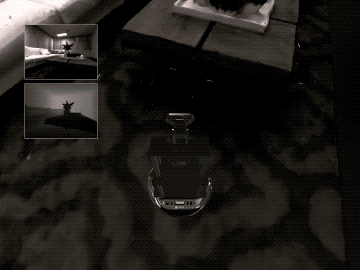## Feature Detail Review

Objects can be instanced from templates (i.e. ObjectAttributes) into the scene by template id with Simulator.add_object() or by template string key with Simulator.add_object_by_handle(). These functions return a unique id which can be used to refer to the object instance. In the case of errors in construction, -1 is returned.

By default, a new SceneNode will be created when an object is instanced. However, the object can be attached to an existing SceneNode (e.g. that of the Agent) if provided. This is demonstrated in Embodied Agents.

Object instances can be removed by id with Simulator.remove_object(). Optionally, the object’s SceneNode can be left behind in the SceneGraph when it is removed (e.g. to prevent deletion of an embodied Agent’s SceneNode).

Simulator.get_existing_object_ids() will return a list of unique object ids for all objects instanced in the scene.

### MotionType

Objects can be configured to fill different roles in a simulated scene by assigning a habitat_sim.physics.MotionType:

### VelocityControl

Each object’s habitat_sim.physics.VelocityControl structure provides a simple interface for setting up continuous velocity control of the object in either the global or local coordinate frame. This can be queried from the simulator with Simulator.get_object_velocity_control().

For habitat_sim.physics.MotionType.KINEMATIC objects, velocity control will directly modify the object’s rigid state.

For habitat_sim.physics.MotionType.DYNAMIC object, velocity control will set the initial velocity of the object before simualting. In this case, velocity will be more accurate with smaller timestep requests to Simulator.step_physics(). Note that dynamics such as forces, collisions, and gravity will affect these objects, but expect extreme damping as velocities are being manually set before each timestep when controlled.